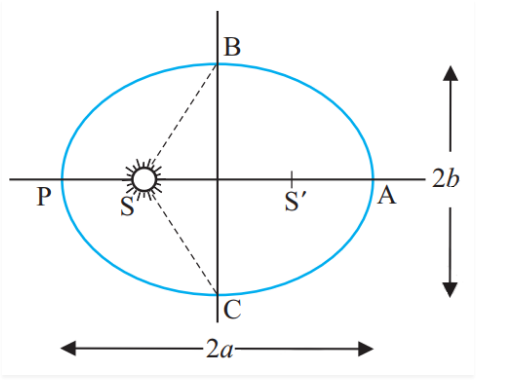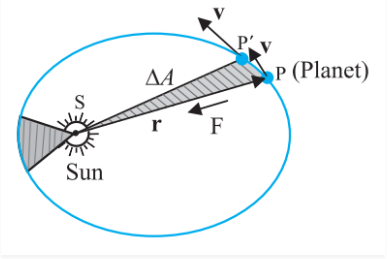# Kepler’s Law MCQs for NEET

Kepler’s law explains the motions of planets in the solar system.

Kepler’s First Law: All the planets move around the sun in elliptical orbits with the sun at the focus.Kepler’s Second Law: The line joining the planet to the sun sweeps out equal areas in an equal interval of time.ΔA is the area swept

Δt is the time interval

Kepler’s Third Law: This law states that the square of the time period (P) is directly proportional to the cube of the semi-major axis of its orbit.

P2 ∝ a3

Q1: When a planet orbits the Sun, one of the foci of the elliptical orbit is

1. The axis
2. The perihelion
3. The centre
4. The Sun

Q2: What has an eccentricity of zero?

1. A straight line
2. A large ellipse
3. A circle
4. A small ellipse

Q3: An Astronomical Unit, or AU, is the average distance between

1. The Sun and Neptune
2. The Sun and Earth
3. The Earth and the Moon
4. The Sun and Mercury

Answer: (b) The Sun and Earth

Q4: Kepler’s second law is known as

1. The Law of Orbits
2. The Law of Areas
3. The Law of Periods
4. The Law of Gravity

Answer: (b) The Law of Areas

Q5: Kepler’s 2nd law deals with

1. The shape of the planet’s orbits
2. The speed/area the planet travels
3. The length of time it takes the planet to orbit the sun
4. Time travel

Answer: (b) The speed/area the planet travels

Q6: Kepler’s third law is known as

1. The Law of orbits
2. The Law of Areas
3. The Law of periods
4. The Law of gravity

Answer: (c) The Law of periods

Q7: The formula for Kepler’s third law is

1. P3 ∝ a2
2. P3 ∝ a3
3. P2 ∝ a2
4. P2 ∝ a3

Q8: Kepler’s first law states that the orbits of the planets are oval in shape or

1. Ellipses
2. Perfect circles
3. Squares
4. triangles

Q9: The Kepler’s first law is known as

1. The law of orbits
2. The law of areas
3. The law of periods
4. The law of gravity

Answer: (a) The law of orbits

Q10: The farther away a planet is from the sun, the …………. it takes it to orbit the sun once

1. Longer
2. Shorter